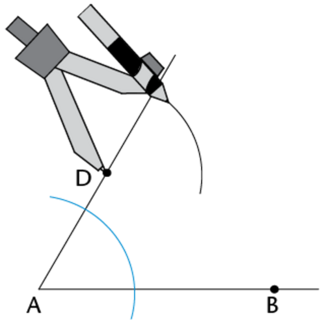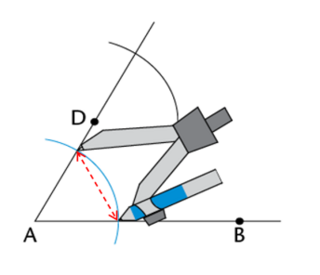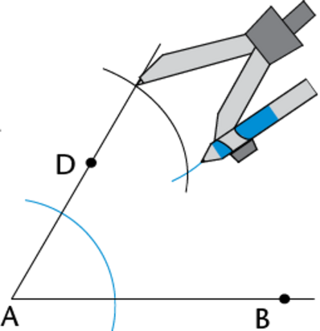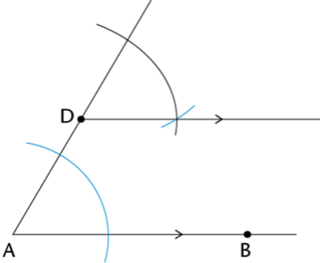Home Practice
For learners and parents For teachers and schools
Textbooks
Full catalogue
Pricing SupportLog in

We think you are located in United States. Is this correct?

# Test yourself now

High marks in science are the key to your success and future plans. Test yourself and learn more on Siyavula Practice.

A quadrilateral is a closed polygon with four straight sides. Examples of quadrilaterals include squares, rectangles, parallelograms and rhombuses. In this section we will construct different quadrilaterals with a compass and ruler.

a closed polygon that has four straight sides

A square is a quadrilateral with all sides equal in length and all interior angles equal to $$90^{\circ}$$.A rectangle is a quadrilateral with opposite sides equal in length and all interior angles equal to $$90^{\circ}$$.A parallelogram is a quadrilateral with opposite sides equal and parallel.A rhombus is a quadrilateral with opposite sides parallel and all sides equal in length.## Worked Example 10.14: Constructing a square

Use a compass and a ruler to construct square $$ABCD$$ with $$AB = 5 \text{ cm}$$.

### Construct $$AB = 5 \text{ cm}$$ and $$\hat{A} = 90^{\circ}$$.

Draw a line segment and mark point $$A$$ on the line. Use a compass to measure a length of $$5 \text{ cm}$$ on your ruler. Keeping the compass width the same, place the compass on point $$A$$ and draw an arc that cuts the line. Label point $$B$$. Use your compass to construct a $$90^{\circ}$$ angle at point $$A$$.### Construct lengths $$AD = 5\text{ cm}$$ and $$BC = 5 \text{ cm}$$.

Use your compass to measure a length of $$5 \text{ cm}$$ on your ruler.

Keeping the compass width the same, place the compass on point $$A$$ and draw an arc that cuts the perpendicular line. Label point $$D$$.

Keeping the compass width the same, place the compass on point $$D$$ and draw an arc above point $$B$$.

Keeping the compass width the same, place your compass on $$B$$ and draw an arc to intersect the other arc above $$B$$. Label the point where the two arcs intersect point $$C$$.### Draw line segments $$BC$$ and $$CD$$.

Use a ruler to draw sides $$BC$$ and $$CD$$ of square $$ABCD$$.

Use your ruler to check the accuracy of the lengths of the sides and confirm that $$AB = BC = CD = DA = 5 \text{ cm}$$.

Use a protractor to measure the interior angles of the square and confirm that $$\hat{A} = \hat{B} = \hat{C} = \hat{D} = 90^{\circ}$$.We can also construct quadrilaterals such as parallelograms and rhombuses using a compass. The following worked example demonstrates how to construct parallel lines.

## Worked Example 10.15: Constructing parallel lines

Use a compass and a ruler to construct a pair of parallel lines.

### Draw line segments $$AB$$ and $$AD$$.

Use a ruler to draw line segments $$AB$$ and $$AD$$. Note that the point $$D$$ will be on the line that will be parallel to $$AB$$. Make sure to extend the line segment $$AD$$ so that there is enough space for the construction.### Construct two arcs at points $$A$$ and $$D$$.

Set your compass to a width that is less than the length of $$AD$$. Place the compass on point $$A$$ and construct a wide arc that intersects $$AB$$ and $$AD$$. Keeping the width of the compass the same, place the compass on point $$D$$ and construct another arc.### Set the compass to the width of the arc intersecting $$AB$$ and $$AD$$.

Set the compass width to the distance between the two points where the first arc crosses $$AB$$ and $$AD$$ (red dotted line).Keeping the compass width the same, place the compass on the point where the second arc crosses $$AD$$ and draw a third arc that intersects the second arc.### Draw a line that passes through $$D$$ and is parallel to $$AB$$.

Draw a line from point $$D$$ and through the point where the two arcs intersect.

This constructed line is parallel to $$AB$$.## Worked Example 10.16: Constructing a parallelogram

Use a compass and a ruler to construct parallelogram $$PQRS$$ with $$PQ = 4 \text{ cm}, QR = 3 \text{ cm}$$ and $$S\hat{P}Q = 45^{\circ}$$.

### Construct $$PQ = 4 \text{ cm}$$ and $$\hat{P} = 90^{\circ}$$.

Draw a line segment and mark point $$P$$ on the line. Use a compass to measure a length of $$4 \text{ cm}$$ on your ruler. Keeping the compass width the same, place the compass on point $$P$$ and draw an arc that cuts the line. Label point $$Q$$.

Use your compass to construct a $$90^{\circ}$$ angle at point $$P$$.### Bisect the right angle at $$P$$.

Use your compass to bisect the right angle at $$P$$ to get $$S\hat{P}Q = 45^{\circ}$$.### Construct $$PS = 3 \text{ cm}$$.

Use your compass to measure a length of $$3 \text{ cm}$$ on your ruler.

Keeping the compass width the same, place the compass on point $$P$$ and draw an arc that intersects the $$45^{\circ}$$ line. Label point $$S$$.

Keeping the compass width the same, place the compass on point $$Q$$ and draw an arc above point $$Q$$.### Construct $$QR$$ and $$RS$$

Use your compass to measure a length of $$4 \text{ cm}$$ on your ruler.

Keeping the compass width the same, place the compass on point $$S$$ and draw an arc that cuts the arc above point $$Q$$. Label the point of intersection $$R$$.

Draw line segments $$QR$$ and $$RS$$.

Use your ruler to check the accuracy of the lengths of the sides and confirm that $$PQ = SR = 4 \text{ cm}$$ and $$QR = PS = 3 \text{ cm}$$.

Use a protractor to measure and confirm that $$S\hat{P}Q = 45^{\circ}$$.Use a compass and a ruler to construct square $$MNPQ$$ with $$MN = \text{4,5} \text{ cm}$$.
Use a compass and a ruler to construct parallelogram $$JKLM$$ using the parallel lines method.
Use a compass and a ruler to construct rectangle $$ABCD$$ with $$AB = 8 \text{ cm}$$ and $$BC = \text{3,5} \text{ cm}$$.
Use a compass and a ruler to construct parallelogram $$WXYZ$$ with $$WX = 7 \text{ cm}$$ and $$XY = 4 \text{ cm}$$ and $$Z\hat{W}X = 60^{\circ}$$.
Use a compass and a ruler to construct rhombus $$PQRS$$ with $$PQ = 6 \text{ cm}$$ and $$S\hat{P}Q = 45^{\circ}$$.
Use a compass and a ruler to construct parallelogram $$QRST$$ with $$QR = 5 \text{ cm}$$ and $$RS = \text{6,5} \text{ cm}$$ and $$T\hat{Q}R = 30^{\circ}$$.